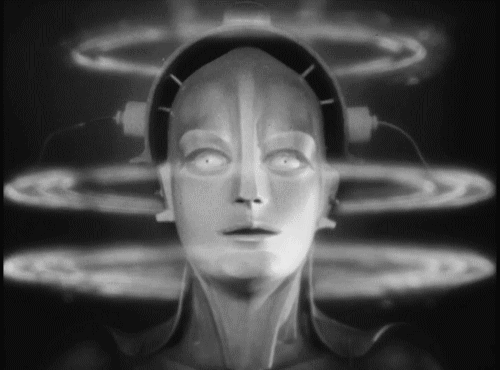# Verification of smart meters

### in IoT environment

by Akim Boyko github / twitter## The Second Machine Age

• 1st Machine Age: computer programmed explicitly, imperative programming
• 2nd Machine Age: machine learning and Big Data: computers learning to program themselves

## The Second Machine Age

• Every time we click at Google/Amazon/etc we taught something about ourselves
• 1/3 of marriages are now from online dating and that number is only increasing. That means that computers (algorithms) are starting to breed humans
• more…

## AI and its applications## AI and its applications

$\begin{bmatrix} y(0) \\ y(1) \\ \ldots \\ y(n) \end{bmatrix} = \begin{bmatrix} 1 & \phi_1(1) & \phi_2(1) & \ldots & \phi_j(1) \\ 1 & \phi_1(2) & \phi_2(2) & \ldots & \phi_j(2) \\ \ldots & \ldots & \ldots & \ldots & \ldots \\ 1 & \phi_1(n) & \phi_2(n) & \ldots & \phi_j(n) \\ \end{bmatrix} \begin{bmatrix} \theta_0 \\ \theta_1 \\ \ldots \\ \theta_n \end{bmatrix}$
$\text{All meters are working as expected} \\ \begin{bmatrix} 15 \\ 27 \\ 39 \\ 11 \\ 8 \end{bmatrix} = \begin{bmatrix} 1 & 10 & 5 \\ 1 & 20 & 7 \\ 1 & 30 & 9 \\ 1 & 10 & 1 \\ 1 & 5 & 3 \end{bmatrix} \begin{bmatrix} \theta_0 \\ \theta_1 \\ \theta_2 \end{bmatrix} \Rightarrow \begin{bmatrix} \theta_0 \\ \theta_1 \\ \theta_2 \end{bmatrix} = \begin{bmatrix} 0.00 \\ 1.00 \\ 1.00 \end{bmatrix}$
$\text{2nd meter measured 10% more} \\ \begin{bmatrix} 14.5 \\ 26.3 \\ 38.1 \\ 10.9 \\ 7.7 \end{bmatrix} = \begin{bmatrix} 1 & 10 & 5 \\ 1 & 20 & 7 \\ 1 & 30 & 9 \\ 1 & 10 & 1 \\ 1 & 5 & 3 \end{bmatrix} \begin{bmatrix} \theta_0 \\ \theta_1 \\ \theta_2 \end{bmatrix} \Rightarrow \begin{bmatrix} \theta_0 \\ \theta_1 \\ \theta_2 \end{bmatrix} = \begin{bmatrix} 0.00 \\ 1.00 \\ 1.11 \end{bmatrix}$
$\text{Network losses 0.05 kWh} \\ \begin{bmatrix} 15.05 \\ 27.05 \\ 39.05 \\ 11.05 \\ 8.05 \end{bmatrix} = \begin{bmatrix} 1 & 10 & 5 \\ 1 & 20 & 7 \\ 1 & 30 & 9 \\ 1 & 10 & 1 \\ 1 & 5 & 3 \end{bmatrix} \begin{bmatrix} \theta_0 \\ \theta_1 \\ \theta_2 \end{bmatrix} \Rightarrow \begin{bmatrix} \theta_0 \\ \theta_1 \\ \theta_2 \end{bmatrix} = \begin{bmatrix} -0.05 \\ 1.00 \\ 1.00 \end{bmatrix}$
$\bullet \text{1st meter measured 5% less} \\ \bullet \text{2nd meter measured 10% more} \\ \bullet \text{Network losses 0.05 kWh} \\ \begin{bmatrix} 15.05 \\ 27.35 \\ 39.65 \\ 11.45 \\ 8.00 \end{bmatrix} = \begin{bmatrix} 1 & 10 & 5 \\ 1 & 20 & 7 \\ 1 & 30 & 9 \\ 1 & 10 & 1 \\ 1 & 5 & 3 \end{bmatrix} \begin{bmatrix} \theta_0 \\ \theta_1 \\ \theta_2 \end{bmatrix} \Rightarrow \begin{bmatrix} \theta_0 \\ \theta_1 \\ \theta_2 \end{bmatrix} = \begin{bmatrix} -0.048 \\ 0.952 \\ 1.111 \end{bmatrix}$

## How Big Data is

• 300MB of raw data per smart meter annually
• 50-2000 smart meters per network segment